# DFF sensor: Transitioning to Inline Rheometery

Drag force flow (DFF) measurement is a new technique for continuous inline powder characterization. Like conventional off-line rheometry, such as dynamic testing, it is based on measurement of the forces associated with the movement of powders or liquids.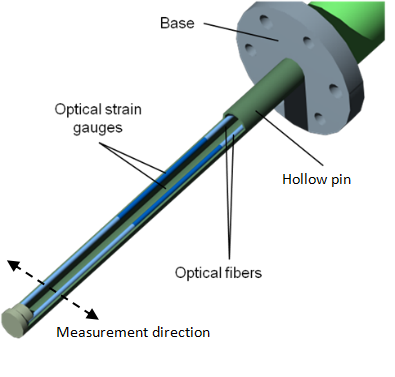## Flow force measurement technology

Lenterra’s inline measurement tool is a needle-like DFF sensor. Flow around it causes a deflection of the tip of the sensor, the magnitude of which is measured with high sensitivity by a pair of optical strain gauges or Fiber Bragg Gratings (FBG) mounted within sensor’s hollow core.

The extent of deflection is directly related to the force exerted on the pin. This force is a function of the properties of the in-process material including the velocity of movement, particle/fluid density and/or viscosity. The evolution of the measured force as a function of time can therefore be correlated directly with process behavior. In addition to force, the optical assembly of the DFF sensor measures temperature of the flow. Information is collected via optical fibers that connect the FBGs within the sensor to an optical interrogator.

DFF sensor can be inserted directly into a vessel or line for continuous powder or liquid flow measurement. The design of the DFF sensor gives it several important advantages for in-process monitoring. For example, measurements are unaffected by electromagnetic interference or the build-up of material on the sensor (fouling), the sensor self-calibrates for temperature and there is no ignition hazard. A measurement rate of 500 Hz provides essentially continuous monitoring enabling the generation of highly repeatable data and the characterization of any periodicity associated with processes such as blending and granulation.

See more detail of the DFF sensor technology in our White Paper 1.### Process characterization metrics: force pulse magnitude (FPM) and pulse consistency factor (PCF)

The figure presents a 1.5-second-long measurement of flow force detected by a DFF sensor inserted into the wet mass during granulation of a placebo formulation in a 10-liter GEA PharmaConnect® granulator. The plot features periodic pulses corresponding to the events of agitator blades periodically passing by the sensor.  Such an evolution provides information about an averaged-in-time flow force (shown by the moving average line) as well as about mass and density of individual granules that manifest themselves as peaks on the plot.

Having information about multiple blade actions collected in a short period of time calls for application of standard statistical methods. The two basic metrics that is calculated from the “raw” measurement are force pulse magnitude (FPM) and pulse consistency factor (PCF).

Values of FPM are obtained by plotting the difference between the highest and lowest values of force measured in a specific time period (see figure).

The time period associated with this calculation can be matched to the process frequency, which is associated with the frequency of rotation of the agitator.

FPM values have are always positive and provide a useful metric for the evaluation of powder characteristics, though useful information can be extracted from an analysis of individual force pulses as well.

FPM values can be used as a rolling average, a common approach in process control. However, it is also possible to derive further metrics for process characterization by using arrays of consecutive FPM values to produce “instantaneous” frequency histograms.

The probability histogram shown in the figure has been generated from 300 FPM data points gathered over a 20-second timeframe.

A log-normal distribution fit can be applied to such histograms to generate two independent parameters: the mean value, MFPM, and the width of the distribution, WFPM.

By advancing in very small increments of time to generate successive, overlapping histograms, as with a moving average, changes in MFPM and WFPM can be determined to monitor the evolution of a process.

In terms of practical significance, MFPM reflects average particle size or density while WFPM is a measure of diversity in the process material, and may be indicative of, for example, the uniformity or cohesiveness in the powder.

These two metrics can be further used to determine a parameter called the Powder Consistency Factor PCF = MFPM/WFPM, which characterizes the uniformity of the flow force. For example, in a system consisting predominantly of fine particles but containing a number of large agglomerates intermittently interacting with the probe, WFPM will be high resulting in a low PCF.

The benefit of using PCF in place of WFPM is that it is a unitless parameter, normalized with MFPM, essentially with particle size.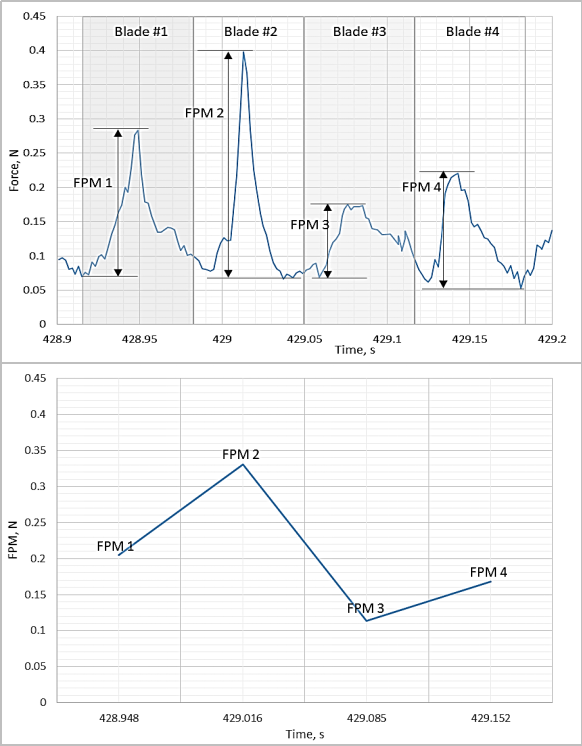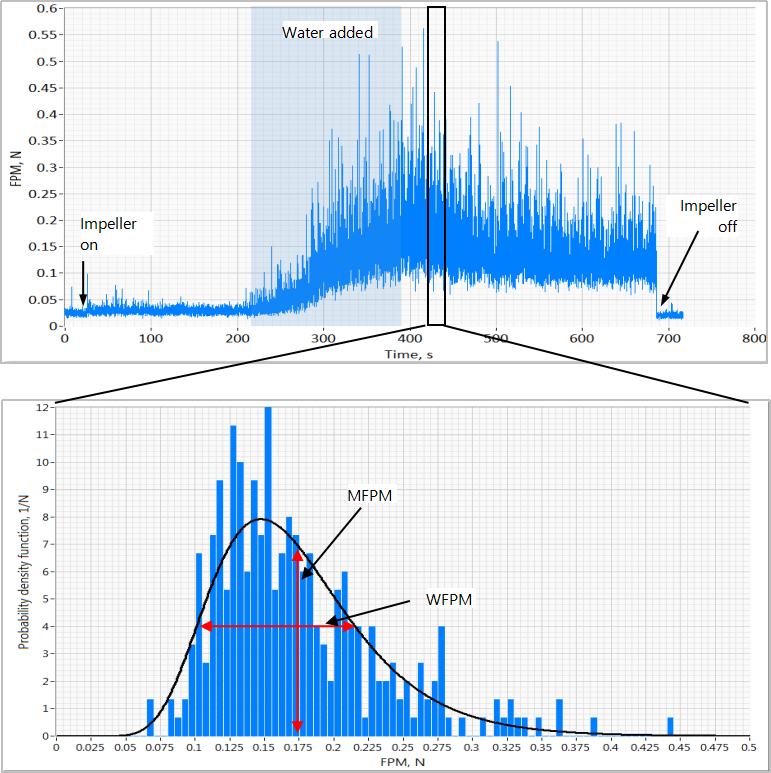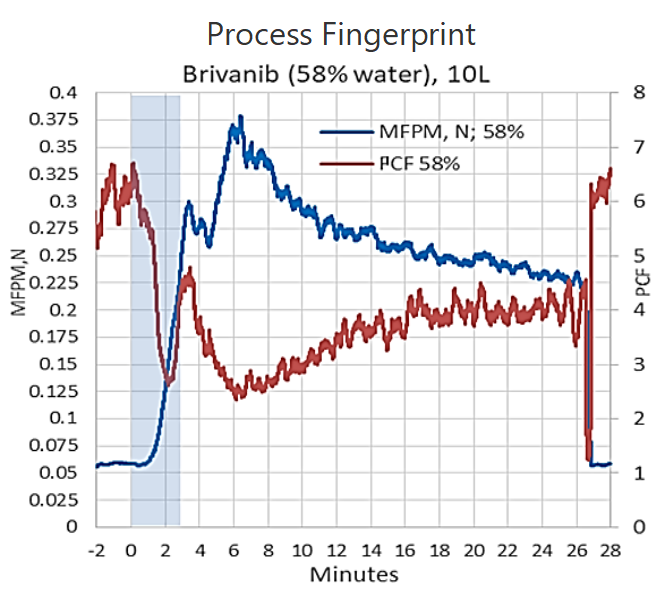Evolutions of MFPM and PCF over the length of the process constitute process signatures. Together MFPM and PCF provide a fingerprint for a powder that characterizes both particle size and density, as well as providing further insight into properties such as cohesiveness.  An example of such a fingerprint for processing Brivanib Alaninate  in a 10L GEA PharmaConnectTM granulator is shown  in the figure. Two characteristic maxima on the FPM signature and the corresponding to them minima in the PCF signature may be used for granulation end point determination and/or scaling.

More information about FPM and PCF metrics is found in our White Papers 3 and 13.

Similarly, FPM and PCF signatures can be used for processing of liquids as in Bio-processing, or for characterization of powders (White Paper 16).

#### Fluid Uniformity

Uniform homogeneous fluid will generate the same values of shear stress from one blade to another which manifest themselves as pulses of the force on the force vs. time evolution. Principal metrics of LIR technology are force pulse magnitude (FPM) and pulse consistency factor (PCF), see detailed description here and our White Papers 3 and 13. Any fluctuation in the fluid density and/or viscosity will result in fluctuation of FPM from one blade to another. Recording several (typically more than 50) FPMs in sequence, one may construct a histogram or distribution of the FPM values. The width of such a distribution would characterize the uniformity of the flow material. A narrow distribution of FPMs indicate high homogeneity and a wider one – a less uniform fluid. This analysis is provided by the LENFLOW measurement software in real time and outputted as PCF (pulse consistency factor). The higher PCF value the more homogeneous fluid material is.

#### Shear stress measurement and estimate of blade shear

DFF sensor probe submerged in a moving fluid gets deflected by the flow as illustrated in the figure. The shear force (friction) on the pin, which is a direct output of the LENFLOW measurement software, is related to the shear stress experienced by the surface of the pin simply as the measured force divided by the surface area of the submerged part of the pin.

.Assuming that flow is uniform along the pin, and accounting for the cylindrical profile of the surface, the averaged wall shear stress is calculated as

τ = \frac {8F} {3As}

where  F is the measured force, As =2πrl  is the surface area of the pin, l is the length of the submerged part of the pin, and r is the outer radius of the pin.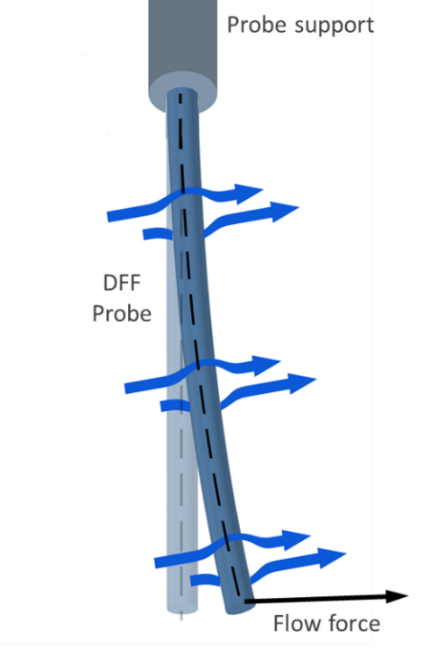In an agitator mixer, the highest flow velocity and therefore shear stresses are produced at the boundary between the blade and the liquid. Similar shear stresses are realized in the boundary layer around the sensor pin installed in the close proximity to the blade as shown in the figure. Then the measurement of the shear stress given by the formula above provides a good estimate of the shear stress experienced by the liquid at the edge of the agitator blade.

#### Viscosity

Connection between the force and viscosity/velocity measurement can be understood as follows. The drag force exerted by the uniform flow on a cylinder depends on the flow velocity as

F = \frac {Cd} {2} Aρυ^2

where Cd is a drag coefficient of a cylinder, A= 2rl is the cross section of the pin, ρ is the fluid density, υ is flow velocity, l is the length of the submerged part of the pin, r  is the outer radius of the pin.

The drag coefficient is a function of the Reynolds number

Re  = \frac {2rυρ} {μ}

where μ is the fluid viscosity. The dependence of drag coefficient on the Reynolds number is well known and graphically represented as

If sensor is in close proximity of the blade (as in figure above) the flow velocity around the probe is close to the velocity of the blade tip, that could be calculated as

υ  = 2πR \frac {RPM} {60}

where R is the blade radius. Then viscosity is calculated as

μ  = rπRρ \frac {RPM} {15Re}

where the value for Reybolds number Re is obtained from the dependence Cd(Re) and Cd is found from the measured force according to the first equation.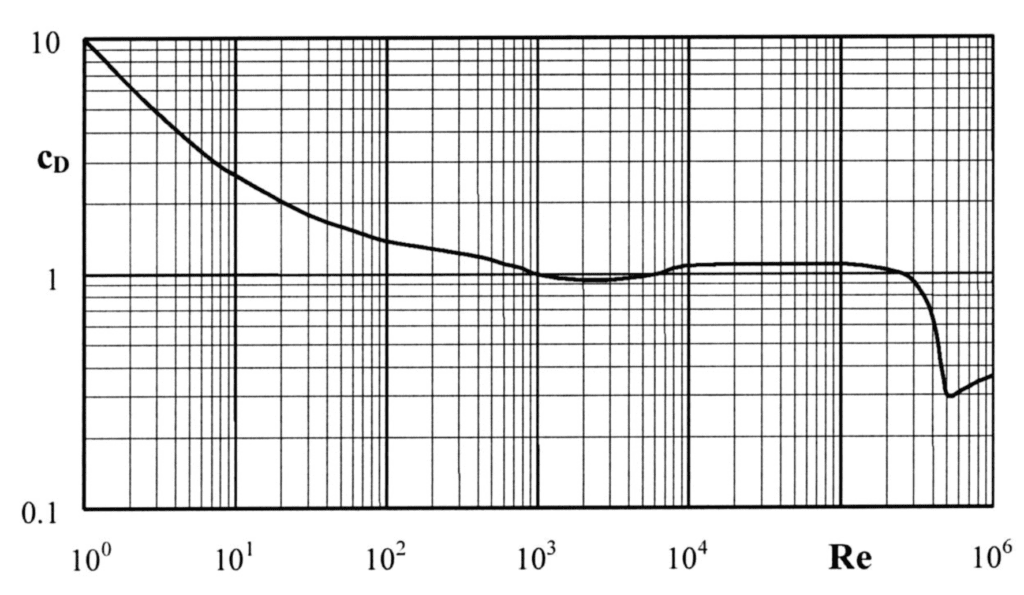#### Temperature Measurement

For both Newtonian and non-Newtonian fluids, viscosity (and thus the fluid velocity and shear rate) is dependent on temperature. For some fluids, even small changes of temperature can result in significant variation in viscosity. As a consequence, accurate interpretation of viscosity measurements should be accompanied by temperature measurements. Lenterra’s sensors are unique in their ability to simultaneously measuring force/shear stress and temperature.